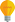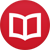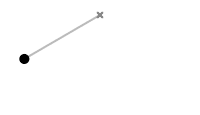# How can chaos be deterministic?

by Jakub MarianTip: See my list of theMost Common Mistakes in English. It will teach you how to avoid mis­takes with com­mas, pre­pos­i­tions, ir­reg­u­lar verbs, and much more.

Perhaps you have already heard the term “deterministic chaos”. It sounds like an oxymoron, doesn’t it? One would expect chaotic behaviour to stem from randomness; people tend to call something that behaves in a very random manner chaotic, but even something random can follow a simple pattern, so randomness alone is not a sufficient condition. In fact, it is not even necessary.

What if a system is deterministic, i.e. its current state completely determines how it will behave in the future? In other words, what if no chance is involved? Can any such system be called chaotic? It turns out there indeed are systems for which this is reasonable. To understand what we mean by “system”, let’s take a look at a system that is not chaotic firsta pendulum:A pendulum is a weight at one end of a string or a rod (or just a rod which itself serves as a weight), the other end of which is firmly attached to something immovable. No sane person would call the motion of a pendulum chaotic; it is one of the simplest and most regular things there are.

Now, imagine a system that is only slightly more complicatedtake a pendulum (consisting of a solid rod) and attach another pendulum (a solid rod) to its end in such a way that it can swing as well. Move the two rods to one side and let them go; this is what happens:The behaviour of the system (called “double pendulum”) becomes extremely erratic, and even though we can observe certain patterns, we can clearly see that the behaviour is what most people would call “chaotic”. How’s that possible? Because the system is extremely sensitive to initial conditions.

## Characterization of deterministic chaos

In a non-chaotic system (such as a simple pendulum), changing the initial state slightly leads to only a slight change in the way the system develops. For example, if the simple pendulum started from a position slightly higher than the one shown here, it would move on an arc that is only slightly longer with a speed that is only slightly larger, but the overall trajectories would look almost the same.

On the other hand, if you changed the initial position of the double pendulum slightly, it would lead to a trajectory that is completely different from the one shown here, and this is the most important property of a chaotic system:

Any change (however small) in the initial state of the system leads to a completely different development of the system after some time.

(There are a few more technical conditions for a system to be called chaotic, but these basically characterize the notion of “being completely different”.) This sensitivity is a more fundamental problem than it might seem at first. Imagine you really construct a double pendulum, set it to some initial position and measure its position. Then run a simulation on your computer (suppose it is completely precise) and release the pendulum at the same time. Within 10 seconds, you will notice that the simulation looks completely different from the real pendulum. So you make your measurements of the pendulum 1000 times more precise and try the process again. It sort of works for 10 seconds, but after 20 seconds, it is completely different again.

However precise you make your measurements, your solution will eventually become completely useless to describe the system. Even worse, we supposed that only the initial conditions were not precise, but in a real simulation, the numerical computation done by a computer would be imprecise as well (you can count with any precision you want, if you have enough computational power, but you can’t count with infinite precision). This means that even two computers computing the same task using similar (but not equal) methods with similar precision would come to different solutions because the “initial conditions” would be slightly different in each step of the simulation.

## Conclusion

While physicists usually work with systems that are not chaotic (for example all devices people normally use work in a non-chaotic manner), the truth is that most systems in nature are chaotic. This in particular means that, even in the realm of classical mechanics, we will in principle never be able to accurately simulate reality in which we live. However precise our measuring devices will be, we will never be able to accurately predict the behaviour of the environment we live in.

By the way, I have written several educational ebooks. If you get a copy, you can learn new things and support this website at the same time—why don’t you check them out?